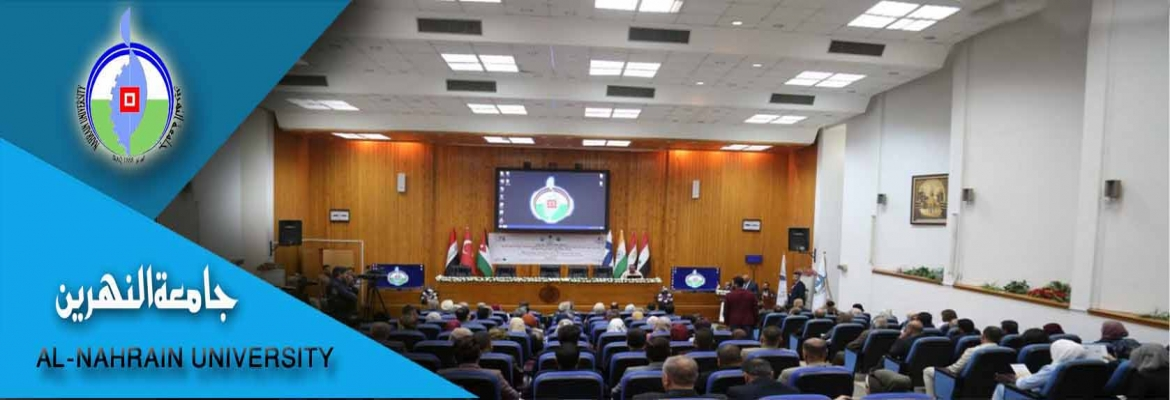# Investigation of some factors affecting the performance of biochemical reactor using MATLAB

number:
2416
Degree:
Author:
Raed Abed Ismael Al-Khazraji
Supervisor:
Dr. Khalid M. Mousa
Assist. Prof. Dr. Basim O. Hasan
year:
2010

In this work, it was attempted to estimate the mass a) and study the dynamic behavior of continuous stirred tank fermenter (CSTF) by means of Matlab language and benefit from commands and functions,which are built in Matlab.transfer coefficient (kL The present work developed a mathematical model for production of biomass as a single cell protein (SCP) by treating backer yeast (biomass) with glucose (substrate 1) and oxygen (substrate 2) as an example for CSTF and used in simulation n programs. This model based on mass balance equations for each of biomass, glucose and oxygen which describe the characteristics of the system, and from which the steady state calculation was obtained and then the stability examined by phase plane technique. Finally,dynamic behavior, could be studied under different RPM and volumes, then different step changes in initial conditions [± 50% and ± 25% step change in feed substrate (S), ± 50% and ± 25% step change in feed dilution rate (D)] were used to study the effect of each on the biomass, substrate and oxygen concentrations, and it found that greatly effect of these changes on the measuring variables.In order to check the stability of our system, phase plane technique was used for the non linear model, and it show that the system is stable.The comparison between experimental results of previous work and theoretical results of non linear model was considered for 1 l working volume (laboratory scale)and 50 l working volume (pilot plant). The results showed that the theoretical non linear was very close to experimental behavior and that give an indication of the accuracy of the present model.From all the theoretical work, it was found that the Matlab programs were suitable and gives the results quickly and accurately.

## اخبار كلية الهندسة

Education - This is a contributing Drupal Theme
Design by WeebPal.